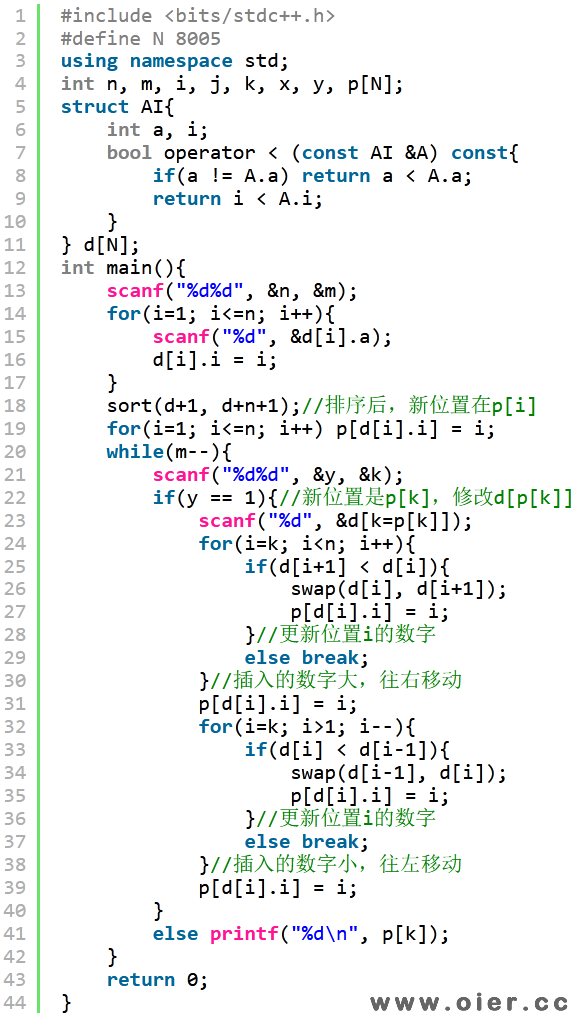325+

“cpp
for (int i = 1; i <= n; i++)
for (int j = i; j >= 2; j–)
if (a[j] < a[j-1]) {
int t = a[j-1];
a[j-1] = a[j];
a[j] = t;
}
“

“pascal
for i:=1 to n do
for j:=i downto 2 do
if a[j]<a[j-1] then
begin
t:=a[i];
a[i]:=a[j];
a[j]:=t;
end;
“

H 老师给了一个长度为 $n$ 的数组 $a$，数组下标从 $1$ 开始，并且数组中的所有元素均为非负整数。小 Z 需要支持在数组 $a$ 上的 $Q$ 次操作，操作共两种，参数分别如下：

$1~x~v$：这是第一种操作，会将 $a$ 的第 $x$ 个元素，也就是 $a_x$ 的值，修改为 $v$。保证 $1 \le x \le n$，$1 \le v \le 10^9$。**注意这种操作会改变数组的元素，修改得到的数组会被保留，也会影响后续的操作**。

$2~x$：这是第二种操作，假设 H 老师按照**上面的伪代码**对 $a$ 数组进行排序，你需要告诉 H 老师原来 $a$ 的第 $x$ 个元素，也就是 $a_x$，在排序后的新数组所处的位置。保证 $1 \le x \le n$。**注意这种操作不会改变数组的元素，排序后的数组不会被保留，也不会影响后续的操作**。

H 老师不喜欢过多的修改，所以他保证类型 $1$ 的操作次数不超过 $5000$。

## 输入输出样例

### 输入样例 #1

3 4
3 2 1
2 3
1 3 2
2 2
2 3


### 输出样例 #1

1
1
2


## 说明

**【样例解释 #1】**

**【样例 #2】**

**【样例 #3】**

**【样例 #4】**

**【数据范围】**

| 测试点 | $n \le$ | $Q \le$ | 特殊性质 |
|:-:|:-:|:-:|:-:|
| $1 \sim 4$ | $10$ | $10$ | 无 |
| $5 \sim 9$ | $300$ | $300$ | 无 |
| $10 \sim 13$ | $1500$ | $1500$ | 无 |
| $14 \sim 16$ | $8000$ | $8000$| 保证所有输入的 $a_i,v$ 互不相同 |
| $17 \sim 19$ | $8000$ | $8000$ | 无 |
| $20 \sim 22$ | $8000$ | $2 \times 10^5$ | 保证所有输入的 $a_i,v$ 互不相同 |
| $23 \sim 25$ | $8000$ | $2 \times 10^5$ | 无 |

## 程序实现